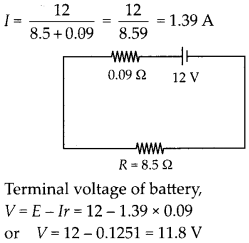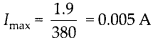Enlightened

# Question 15: NCERT Solutions for 12th Class Physics: Chapter 3-Current Electricity

• 0

(a) Six lead-acid type of secondary cells each of emf 2.0 V and internal resistance of 0.015 Ω are joined in series to provide a supply to a resistance of 8.5 Ω. What are the current drawn from the supply and its terminal voltage?

(b) A secondary cell after long use has an emf of 1.9 V and a large internal resistance of 380 Ω. What maximum current can be drawn from the cell ? Could the cell drive the starting motor of a car ?

Share

1. Solution:
(a) Six cells are joined in series.Equivalent emf is 2 × 6 = 12 V
Equivalent internal resistance is 0.015 × 6 = 0.09 Ω
Current drawn from supply(b) Maximum current is drawn from a battery when external resistance is tested to be zeroTo start a car, a current of the order of 100 A is needed, so the battery mentioned above can not drive the starting motor.

Check the complete chapter with solutions.

NCERT Solutions for 12th Class Physics: Chapter 3-Current Electricity

• 0